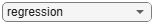# Basic Loss Given Default Model Validation

This example shows how to perform basic model validation on a loss given default (LGD) model by viewing the fitted model, estimated coefficients, and p-values. For more information on model validation, see `modelDiscrimination` and `modelCalibration`.

```load LGDData.mat head(data)```
``` LTV Age Type LGD _______ _______ ___________ _________ 0.89101 0.39716 residential 0.032659 0.70176 2.0939 residential 0.43564 0.72078 2.7948 residential 0.0064766 0.37013 1.237 residential 0.007947 0.36492 2.5818 residential 0 0.796 1.5957 residential 0.14572 0.60203 1.1599 residential 0.025688 0.92005 0.50253 investment 0.063182 ```

### Fit Model and Review Model Goodness of Fit

Create training and test datasets to perform a basic model validation.

```rng('default'); % for reproducibility NumObs = height(data); c = cvpartition(NumObs,'HoldOut',0.4); TrainingInd = training(c); TestInd = test(c);```

Fit the model using `fitLifetimePDModel`.

```ModelType ="regression"; lgdModel = fitLGDModel(data(TrainingInd,:),ModelType,... 'ModelID','Example',... 'Description','Example LGD regression model.',... 'PredictorVars',{'LTV' 'Age' 'Type'},... 'ResponseVar','LGD'); disp(lgdModel)```
``` Regression with properties: ResponseTransform: "logit" BoundaryTolerance: 1.0000e-05 ModelID: "Example" Description: "Example LGD regression model." UnderlyingModel: [1x1 classreg.regr.CompactLinearModel] PredictorVars: ["LTV" "Age" "Type"] ResponseVar: "LGD" ```

Display the underlying statistical model. The displayed information contains the coefficient estimates, as well as their standard errors, t-statistics and p-values. The underlying statistical model also shows the number of observations and other fit metrics.

`lgdModel.UnderlyingModel`
```ans = Compact linear regression model: LGD_logit ~ 1 + LTV + Age + Type Estimated Coefficients: Estimate SE tStat pValue ________ ________ _______ __________ (Intercept) -4.7549 0.36041 -13.193 3.0997e-38 LTV 2.8565 0.41777 6.8377 1.0531e-11 Age -1.5397 0.085716 -17.963 3.3172e-67 Type_investment 1.4358 0.2475 5.8012 7.587e-09 Number of observations: 2093, Error degrees of freedom: 2089 Root Mean Squared Error: 4.24 R-squared: 0.206, Adjusted R-Squared: 0.205 F-statistic vs. constant model: 181, p-value = 2.42e-104 ```

In the case of the underlying statistical model for a `Regression` model, the underlying model is returned as a compact linear model object. The compact version of the underlying `Regression` model is an instance of the `classreg.regr.CompactLinearModel` class. For more information, see `fitlm` and `CompactLinearModel`.